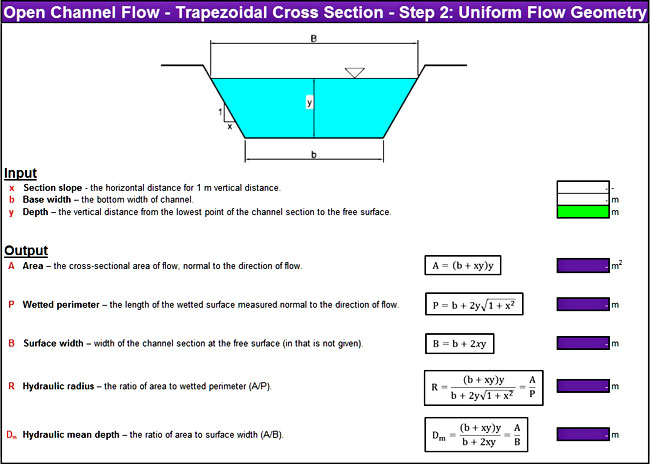# Open Channel Flow Trapezoidal Section Calculator In Excel

May 13, 2011### Introduction

Here is another freeware tool that I developed in Excel. With this workbook, you can calculate the discharge, the velocity, the Reynolds number, and the type of flow (laminar, transitional, turbulent) of an open channel with a trapezoidal cross-section. Manning’s equation is used for the calculations.

### How to use the open channel flow trapezoidal section calculator

There are three discrete steps:

• First, go to the first sheet named “Step 1” and fill the channel’s geometry. If you have an unknown value, the sheet will calculate it.
• Then, go to “Step 2” and fill the channel’s depth. Automatically, the sheet will calculate some necessary parameters, such as wetted perimeter, hydraulic radius, and others.
• Finally, go to “Step 3” and fill the values in the green cells. Two useful tables will help you fill the Manning’s parameter, as well as the dynamic viscosity of water. If you have correctly filled all the input values, this sheet will calculate the flow’s desired values (discharge, velocity, and type of flow).

The workbook can be opened with Excel 2007 or newer. Please, enable macros before using it.

Open Channel Flow – Rectangular Cross Section

Category: Freeware Tools